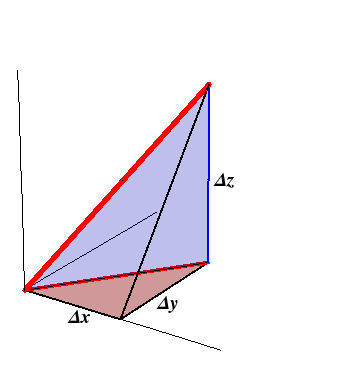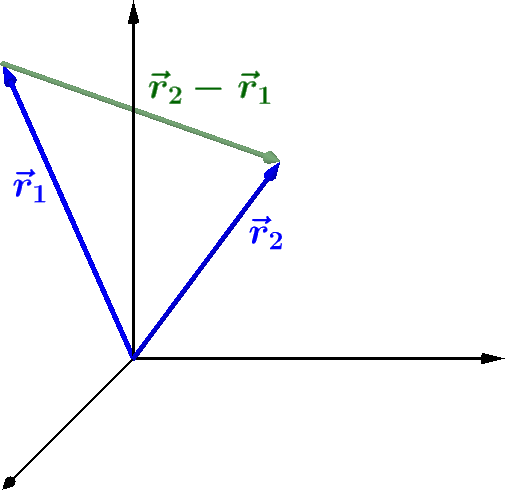## Section1.16The Distance Formula

If you want to find the distance between two objects in the real world, you measure the distance with a ruler (unless you are an astrophysicist and the distances are too large or you are a particle physicist and the distances are too small!). But, if you are a theoretician and you want to put the distance into a formula, things are not quite so simple. First, you need information about where the two objects are. Perhaps you know coordinates of the two points in some rectangular coordinate system, say $$(x_1, y_1, z_1)$$ and $$(x_2, y_2, z_2)\text{.}$$ Then the three-dimensional version of the Pythagorean theorem shows that the distance between the objects is

\begin{align} \hbox{distance} \amp= \sqrt{(x_2-x_1)^2 +(y_2-y_1)^2 +(z_2-z_1)^2} \\\notag\\ \amp= \sqrt{(\Delta x)^2+(\Delta y)^2+(\Delta z)^2} .\tag{1.16.1} \end{align}

Use the Pythagorean Theorem in 2 dimensions to derive formula (1.16.1) for the Pythagorean Theorem in 3 dimensions.

Hint.

To determine the length of the heavy red line, first project it into the $$xy$$-plane. Use the Pythagorean theorem twice, first on the (projected) red triangle and then on the (vertical) blue triangle in Figure 1.16.2.Figure 1.16.2. The Pythagorean theorem can be extended to three dimensions.

But what if the theoretician wants to use a coordinate independent expression in her formulas. How does she say where the objects are? By using the position vectors $$\vec{r}_1$$ and $$\vec{r}_2\text{.}$$ Figure 1.16.3 shows the position vectors $$\vec{r}_1$$ and $$\vec{r}_2$$ for two objects, as well as their difference, $$\vec{r}_2-\vec{r}_1\text{.}$$ The magnitude of this latter vector is the distance between the two objects. The magnitude property of the dot product says that the magnitude of any vector is the square root of the dot product of the vector with itself. Therefore, the distance between the two objects, in coordinate independent form is:

\begin{equation} \hbox{distance} = \vert \vec{r}_2-\vec{r}_1\vert = \sqrt{(\vec{r}_2-\vec{r}_1)\cdot (\vec{r}_2-\vec{r}_1)} .\tag{1.16.2} \end{equation}Figure 1.16.3. The position vectors $$\vec{r}_1$$ and $$\vec{r}_2$$ for two objects, and their difference, $$\vec{r}_2-\vec{r}_1\text{.}$$ The magnitude of the difference is the distance between the two objects.